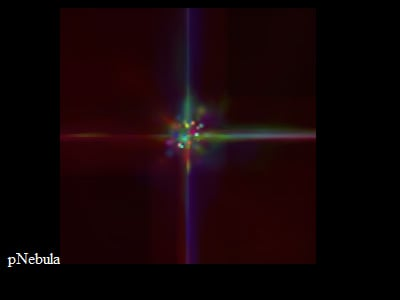# PNEBULA

111 wordsMathieu 'p01' Henri on September 30th, 2008

# pNebula

Almost the first 256b intro using Canvas ever :pThe idea was pretty simple: Draw colorful dots in the center of the Canvas and zoom it all introduce a nebula like color blending.

``<body bgcolor=0 text=snow onload=h=R.height=256;setInterval('with(R.getContext("2d"))fillStyle="#"+(999*r(fillRect(r(drawImage(R,3,3,250,250,0,0,h,h))*26+116,r()*26+116,3,3))|0)',r=Math.random)>pNebula<canvas id=R>``

Including some styling of the page and text, the whole intro fits in 214 bytes. Actually it was even possible to fit a valid HTML5 version in 248 bytes.

``<!doctype html><title></title>pNebula<canvas></canvas><script>r=Math.random;setInterval('with(R.getContext("2d"))fillStyle="#"+(999*r(fillRect(r(drawImage(R,3,3,144,144,0,0,150,150))*26+61,r()*26+61,3,3))|0)',R=document.body.childNodes)</script>``